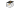The contents of all tutorial videos

Tutorial contentsTutorial 1: Basic interactions and viewing options

• Basic interactions

• Visualising object elements

• Selecting and deselecting

• Dragging with Shift (perpendicular)

• Undo / redo / refresh

• Pan, Zoom

• View from different sides

Tutorial 2: Edge Design

• Adding vertices at edge fractions

• Adding vertices on multiple edges

• Adding vertices around a face

• Adding vertex across from vertex

• Adding straight edges with shift

• Drawing edges on faces

• Drawing straight edges with shift-E

• Adding vertices while drawing edges

• Carving

• Splitting faces

• Offsetting faces

• Tooltips and Help

• Floating context toolbar

Tutorial 3: More Viewing Options, Colors and Materials

• Viewing perpendicular to face

• Storing and retrieving viewpoints

• Coordinate axes and planes

• Displaying grid

• Displaying vertex coordinates (moving / selected / all)

• Setting face colors

• Setting face materials

• Viewing triangulated mesh

Tutorial 4: Pop-outs, Holes and Bridges

• Pop-out

• Pop-in

• Pop-out-cut (thicken)

• Making holes through bodies

• Connecting bridges

• Stitch

Tutorial 5: Moving, Scaling and Dimensioning

• Moving and rotating elements

• Global and local positioning

• Dragging multiple vertices

• Place manipulator at vertex

• Repositioning the manipulator

• Snapping to body

• Live scaling

• Scaling objects with precision

• Setting the scaling center

• Scaling based on distance

• Scaling non-proportionately

• Setting precise values

• Set single vertex coordinates with shift-click

• Setting multiple vertex coordinates

Tutorial 6: Fixing, Deleting and Dissolving

• Fixing objects

• Unfixing objects

• Deleting edges or vertices

• Deleting a vertex with more than 2 edges

• Making faces invisible

• Filling invisible faces

• Dissolving faces into one

• Dissolving to split body in two

Tutorial 7: Face and Edge Shapes

• Flattening faces

• Flattening multiple faces

• Flattening edges

• Making edges linear

• Making edges collinear

• Making faces or edges circular

• Turning edges into circular arcs

• Making circles with precision

• Copying edges

Tutorial 8: Smoothness and Continuity

• Smoothing edges

• Setting smoothen strength

• Removing edge smoothness

• Smoothing faces

• Smoothing edge with respect to a face

• Curvature combs

Tutorial 9: Mirroring and Symmetries

• Mirroring model

• Mirror planes

• Setting symmetry plane

• Forming symmetry sets

• Clearing symmetry

• Mirror vs. Symmetry

• Combining Mirror and Symmetry

Tutorial 10: Dimensioning and Precision

• Setting the dimensions of the initial block

• Setting the distance between two vertices (and choosing the effect on the entire body)

• Displaying and Setting Coordinates

• Using the Grid

• Adding vertices on fractions of an edge

• Adding a vertex across an existing one

Tutorial 11: Multiple Bodies

• Copying one body, or more at once

• Copied bodies are independent

• Merging two bodies into one

• Splitting a body in two

• Snapping one body onto another

Tutorial 12: Load, Save, Import and Export

• Phi: A connected Onshape App

• Saving in the Phi format

• Save Phi model in Onshape document

• Auto-saving

• Sending to Onshape Part Studio

• Exporting to STEP or STL

• Bring in model from Onshape Part Studio

• Load a saved Phi model

• Bringing in model from Onshape Part Studio: known issues and workarounds

Tutorial 13: Reference Objects and Reference Sketches

• Importing reference objects

• Hiding reference objects

• Editing reference objects

• Snapping onto reference object

• Convert reference faces to Phi faces

• Importing reference STL files

• Importing reference sketches

• Default reference sketches

• Editing reference sketches

Tutorial 14: Settings, Help and Feedback

• Show or hide labels

• Disable tooltips

• Set workspace units

• Set coordinate decimals

• Set smoothen strength (and break tangency flag)

• Curvature combs

• Inner side red

• Consistency checks

• Grid spacing

• Backgrounds and lighting

• Set display triangles per face

• Start or stop autosave

• Reference model appearance

• Search commands

• Floating toolbar

• Tutorial videos

• Feedback button

© 2022 Phenometry P.C. branded by